Basic Principles of Anomalous Scattering and MAD

Professor John R Helliwell
Department of Chemistry
University of Manchester
M13 9PL, U.K.

1. Introduction

The phase problem in macromolecular crystallography is amenable to the multiwavelength anomalous dispersion method, which is now known as the MAD method. The theory of various approaches has been developed over a long period (see e.g. Okaya and Pepinsky (1956), Mitchell (1957), Herzenberg and Lau (1967), Karle (1967, 1980), Hoppe and Jakubowski (1975)). The harnessing of synchrotron radiation and the chance thereby to finely tune the X-ray wavelength around the X-ray absorption edge of a target (heavy) atom, so as to vary the scattering in amplitude and phase of that atom, has made the method practical. If an anomalous scatterer can certainly be introduced, as with the seleno-methionine labelling of proteins (Hendrickson (1991)), then relatively speedy structure determination of a new protein structure is practicable. The protein size that is tractable is advancing as synchrotron machine, and beamline, as well as detector capability, improves. Experimental knowledge of anomalous dispersion effects has also improved. This involves fundamental studies whereby very fine profiles (Arndt et al. (1982)) or anisotropies (Templeton and Templeton (1985)) have been investigated for bound atoms. Moreover different strategies in macromolecular crystal phase determination (Peterson et al. (1996)) as well as phase improvement and extension methods (Chayen et al. (1996)) have given practical ideas of these capabilities. For other examples see the companion papers in this Proceedings. The growth of the number of SR instruments in the last 15 years is testimony to a confidence in these approaches. For an overview see Helliwell (1992) and for an update see Chayen et al. (1996), as well as ideas and plans at the outset see Helliwell (1979). The purpose of this paper is to set down the principles and basis of anomalous scattering for MAD.

2. X-ray absorption and scattering

An electron of an atom can be ejected when a photon has sufficient energy. A heavy atom has K and L, or even M, edges in the wavelength range which is useful for X-ray crystallography. The atomic scattering factor for X-rays of that atom in the resonant condition becomes complex i.e. alters the normal scattering factor in amplitude and phase. The anomalous dispersion coefficients f' and f" are used to describe this effect and which are wavelength dependent. Hence for the heavy atom we have(1)

This equation thereby serves to correct for the standard, more simple, model of X-ray scattering. 'Normal scattering' is basically determined by the total number of electrons in the atom and which takes no account of absorption edge resonance effects. For a heavy atom this is not the situation for the wavelengths we use! For the light atoms (C, N, O and H) their corrections to the normal scattering are negligible for our purposes. A free atom (i.e. without neighbours) has a relatively simple form for the variation with wavelength of f' and f" (figure 1a). The edge wavelength is then where the scattering factor becomes complex. A bound atom has neighbours which can scatter back the ejected photoelectron and thereby seriously modulate the absorption effect and also alter therefore the X-ray scattering anomalous dispersion coefficients (figs. 1b and c). Furthermore the values can become dependent on direction (so called dichroism effects) as there can be for example a high density of neighbours in one direction or plane over another (for example see Templeton and Templeton (1985) for). Moreover the exact edge position depends on the oxidation state of the atom as the inner shell electron can be more tightly bound when valence outer shell electrons are removed. Finally the X-ray wavelength bandpass needs to be considered because inherent effects can be masked if this is broader than the linewidths of the features naturally present. To guide our understanding of these phenomena the classical treatment of the 'forced harmonic oscillator with damping' will be reviewed in the next section as it forms the basis for the free atom case.

3. A mathematical model: Forced harmonic oscillator with damping

An inner shell electron can be modelled as having vibrational motion in an alternating electric field subject to a restoring force with associated spring constant k and a damping force proportional to the velocity (see Woolfson (1970, 1997)). The equation of motion is then described by the equation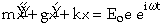(2)

Since we can expect that the oscillation of the electron will not in general be in phase with the driving electric field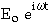then the amplitude will prove to be complex. Hence the amplitude and phase angle between the driving force and the resultant oscillation must be considered. The undamped resonant frequency is given by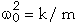(3)

The amplitude solution to the forced damped case is then(4)

Hence the modulus of the amplitude is obtained by multiplying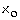by its complex conjugate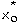and taking the square root to give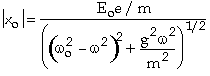(5)

and the phase angle of the oscillation relative to the driving force is then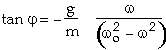(6)

Equations 5 and 6 tell us much about the basic resonance condition. This is obviously according to the mechanical oscillator model rather than where, at resonance, the electron is ejected as a photoelectron! So this is basically referred to as the classical treatment rather than the full quantum treatment. One aspect of the approximation then which is incorrect is that the imaginary part of the complex amplitude varies symmetrically about the resonance frequency but of course f", which is the analogous quantity, is only finite on one side of the frequency range (namely the high frequency, short wavelength range in the atomic case). Nevertheless a plot of the amplitude and phase versus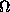, for various degrees of damping (expressed numerically as the Q factor of the resonance where) shows that the resonance when free of damping (infinite Q) is instantaneous. At finite Q the resonance is not sharp and can be characterised by the frequency band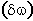over which the amplitude has a valueof the resonant amplitude. In fact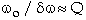(7)

In the case of radiation, rather than a mechanical oscillator, the damping effect is usually attributed to radiation damping, which is weak and thereby Q is very large and the resonance is very sharp (see figure 1a) and whereby the core hole lifetime defines the line width. Note also that if the application of the electric field involves a frequency bandpass then this will degrade the resonance sharpness measured experimentally.

At low Q there is a marked difference between the frequency for maximum amplitude when undamped than when it is damped i.e.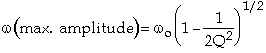(8)

In this treatment, as well as the limitation of ignoring the ejection of the photo-electron, we are assuming that the atom is a free atom and that of course is not true in the bound atom case.

4. X-ray anomalous dispersion coefficients and the KKT

The quantities f' and f" are related by the Kramers-Kronig transform (KKT for short). These are(9a)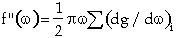(9b)

Since the mass absorption coefficient is proportional to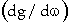(i.e. f") the measured absorption or fluorescence spectrum from the sample can be used to derive f' also.

These equations give the minimum of f' at the half way point up the edge i.e. f" for the simple step function absorption edge case (note that this is not as shown in the paper Hoppe and Jakubowski (1975) page 445 figure 4 which places f' min at f" max and which is correct for a resonance of infinite Q; see also the tabulated theory cases i.e. as per Sasaki (1989) which is similar for the interval used of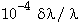5. Maximal dispersive and anomalous signals in the 'white line' case

The presence of 'white line' features alters the simple edge shape (fig. 1b and c); in such a situation 'half way up' the edge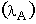is between the floor of the pre-edge region and the top of the spike and is the f' minimum; the top of the absorption spike is a value of f' mid way between f' min and f' maxthe point half way down the absorption spike to the post edge plateau is an f' maximum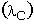. Clearly the maximal Æf' and a maximal f" to be stimulated can be probed by use of the wavelengths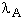and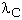as well asrespectively. Use ofandalone, whilst yielding maximal Æf' would (via) involve a slight reduction in size of f" harnessed.

6. Concluding remarks and the scope of the method

Anomalous scatterers can now be purposely inserted into a protein such as seleno-methionine labelling (Hendrickson (1991)) or the wide range of accessible absorption edges for different types of metal atom derivative utilised. Anomalous dispersion effects are optimised when the X-ray wavelength is tuned right to an absorption edge. Further enhancements are possible experimentally (for a recent review see Chayen et al. (1996)) by use of high brilliance insertion devices such as tunable undulators, in conjunction with high heat load optics, whereby a high flux onto the sample is preserved even with a tiny bandpass (i.e. 10-5 to 10-4) so as to explore sharper linewidth features and possibly dichroism as well. Moreover hybrid experimental strategies involving a 'quick pass' data collection at a minimum of two wavelengths followed by a 'slow pass' higher resolution data collection at one wavelength could allow novel theoretical atomic resolution direct methods to be combined with the MAD methods. These then are the sort of structure determination tools that can form the basis to tackle the tens of thousands of protein structures of interest on genome scales.

References

Arndt, U.W., Greenhough, T.J., Helliwell, J.R., Howard, J.A.K., Rule, S.A. and Thompson, A.W. Nature, 298 (1982) 835-838.

Chayen, N.E., Boggon, T.J., Cassetta, A., Deacon, A., Gleichmann, T., Habash, J., Harrop, S.J., Helliwell, J.R., Nieh, Y.P., Peterson, M.R., Raftery, J., Snell, E.H., Hadener, A., Niemann, A.C., Siddons, D.P., Stojanoff, V., Thompson, A.W., Ursby, T. and Wulff, M. Quarterly Reviews in Biophysics, 28 (1996) 227-278.

Helliwell, J.R. Daresbury Study Weekend Proceedings DL/SCI, R13 (1979) p1-6.

Helliwell, J.R. Reports on Progress in Physics, 47 (1984) 1403-1497.

Helliwell, J.R. "Macromolecular Crystallography with Synchrotron Radiation" Cambridge University Press (1992).

Hendrickson, W.A. Science, 254 (1991) 51-58.

Herzenberg, A. and Lau, H.S.M. Acta Cryst, 22 (1967) 24-28.

Hoppe, W. and Jakubowski, U. in Anomalous Scattering. Edited by Abrahams, S.C. and Ramaseshan, S. (1975) pp 437-461.

Karle, J. Applied Optics, 6 (1967) 2132-2135.

Karle, J. Int. J. of Quantum Chemistry, 7 (1980) 356-367.

Mitchell, C.M. Acta Cryst, 10 (1957) 475-476.

Okaya, Y. and Pepinsky, R. Phys. Rev., 103 (1956) 1645.

Peterson, M., Harrop, S.J., McSweeney, S.M., Leonard, G.A., Thompson, A.W., Hunter, W.N. and Helliwell, J.R. J. Synchrotron Rad., 3 (1996) 24-34.

Sasaki, S. KEK Report (1989) 88-14. National Laboratory for High Energy Physics, Tsukuba, Japan.

Templeton, D.H. and Templeton, L.K. Acta Cryst, A41 (1985) 365-371.

Woolfson, M.M. X-ray crystallography (1970) [2nd Edition 1997] Cambridge University Press.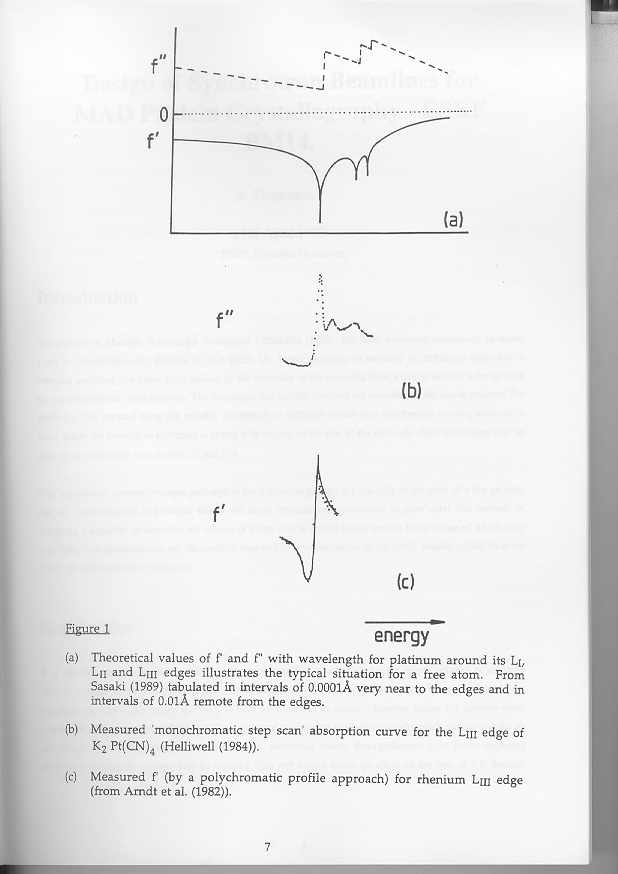Figure 1

(a) Theoretical values of f' and f" with wavelength for platinum around its LI, LII and LIII edges illustrates the typical situation for a free atom. From Sasaki (1989) tabulated in intervals of 0.0001 very near to the edges and in intervals of 0.01 remote from the edges.

(b) Measured 'monochromatic step scan' absorption curve for the LIII edge of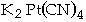(Helliwell (1984)).

(c) Measured f' (by a polychromatic profile approach) for rhenium LIII edge (from Arndt et al. (1982)).